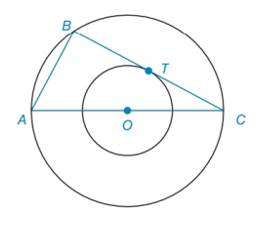Chapter 6.2, Problem 48E### Elementary Geometry for College St...

6th Edition
Daniel C. Alexander + 1 other
ISBN: 9781285195698

#### Solutions

Chapter
Section### Elementary Geometry for College St...

6th Edition
Daniel C. Alexander + 1 other
ISBN: 9781285195698
Textbook Problem
1 views

# Given concentric circles with center O , Δ A B C is inscribed in the larger circle, as shown. If B C ¯ is tangent to the smaller circle at point T and A B = 8 , find the length of the radius of the smaller circle.To determine

To find:

The length of the radius of the smaller circle.

Explanation

Calculation:

Given, concentric circles with center O.

ΔABC is inscribed in the larger circle as shown below.

It is also given that, BC¯ is tangent to the smaller circle at point T and AB=8.

Now draw a line joining O and T.

OT is the radius of smaller circle and OA is the radius of the bigger circle.

So, OTCT.

OTC=90°.

From the figure, ABBC.

So, ABC=90°

Therefore,

OTC=ABC

And again from the figure,

OCT=ACB

Hence, ΔOTCΔABC by AA similarity

### Still sussing out bartleby?

Check out a sample textbook solution.

See a sample solution

#### The Solution to Your Study Problems

Bartleby provides explanations to thousands of textbook problems written by our experts, many with advanced degrees!

Get Started

#### Evaluate the expression sin Exercises 116. (2)3

Finite Mathematics and Applied Calculus (MindTap Course List)

#### Find the domain of the function. a. f(x) = 9x b. f(x) = x+32x2x3

Applied Calculus for the Managerial, Life, and Social Sciences: A Brief Approach

#### Evaluate the definite integral. 01ez+1ez+zdz

Single Variable Calculus: Early Transcendentals

#### Rewritten in reverse order,

Study Guide for Stewart's Multivariable Calculus, 8th

#### The general solution to (for x, y > 0) is: a) y = ln x + C b) c) y = ln(ln x + C) d)

Study Guide for Stewart's Single Variable Calculus: Early Transcendentals, 8th